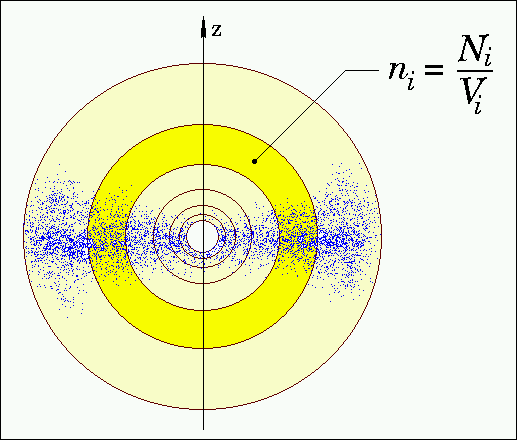Manual - Upload: Density Distribution. N-Particle Simulation.If you are planning to upload a dust density distribution resulting from n-particle simulations you may proceed as follows:
1. Subdivide your model space into spherical shells with decreasing shell thickness towards the center in order to provide a well defined density structure in the region with the highest temperature gradient. Furthermore, make sure that the subdivision of the model space traces the most significant features of your density distribution (gaps, local maxima etc.).
2. Derive the number density of grains n within all volumes V (spherical shells centered on the illuminating star) based on the number of particles N within that volume. The corresponding radii should chosen within this volume (i.e., the arithmetic mean of the inner and outer radius of each shell).
3. Let n(1) and n(100) be the number densities at the innermost and outermost shell of a model subdivided into 100 shells. Let r(1) and r(100) be the corresponding  radii. Then, your file prepared for upload of the density distribution should have the following structure:
 # Some comments....... ...... (if you like) 102 # Number of entries, here: Number of shells +2 Inner radius [AU] n(1) r(1)[AU] n(1) r(2) [AU] n(2) ... r(99) [AU] n(99) r(100) [AU] n(100) Outer radius [AU] n(100)

If particles are present within the sublimation radius of the particular type of dust, they are removed from the density distribution. In this case, the mass of the shell will normalized to the user-defined value based on the remaining dust outside the sublimation region.

Hint: As outlined in the general remarks concerning uploaded density distributions , a grid with logarithmic equidistant spacing may be a good choice in case of smooth density distributions but not in case of small structures (gaps/clumps/holes) present at large distances from the star.